Courses

# Test: Introduction To Graphs- 3

## 20 Questions MCQ Test Mathematics (Maths) Class 8 | Test: Introduction To Graphs- 3

Description
This mock test of Test: Introduction To Graphs- 3 for Class 8 helps you for every Class 8 entrance exam. This contains 20 Multiple Choice Questions for Class 8 Test: Introduction To Graphs- 3 (mcq) to study with solutions a complete question bank. The solved questions answers in this Test: Introduction To Graphs- 3 quiz give you a good mix of easy questions and tough questions. Class 8 students definitely take this Test: Introduction To Graphs- 3 exercise for a better result in the exam. You can find other Test: Introduction To Graphs- 3 extra questions, long questions & short questions for Class 8 on EduRev as well by searching above.
QUESTION: 1

### Which of the following statements is true?

Solution:

Origin is the point of intersection of the X and Y axes.

QUESTION: 2

### Which of the points given is a point on the X-axis?

Solution:

A point on the X-axis has its y-coordinate zero 0.

QUESTION: 3

### Direction: The bar-graph provided gives the sales of books (in thousand numbers) by six branches of a publishing company during two consecutive years 2013 and 2014. Sales of books (in thousand numbers) in different branches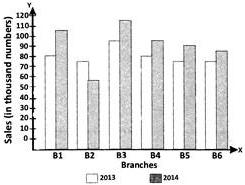What is the total sales of branches B1, B3 and B5 together for both the years (in thousand numbers)?

Solution:

Total sales of branches B1,B3, and B5 together for both the years (in thousand numbers)
=(80+105)+(95+115)+(785+95) = 565

QUESTION: 4

The bar-graph provided gives the sales of books (in thousand numbers) by six branches of a publishing company during two consecutive years 2013 and 2014. Sales of books (in thousand numbers) in different branches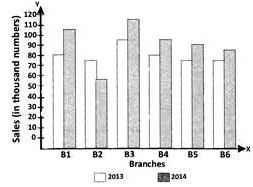What percent of the total sales of branch B3 for both the years is the total sales of branch B6 for both the years?

Solution:

The required percentage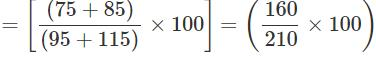QUESTION: 5

The bar-graph provided gives the sales of books (in thousand numbers) by six branches of a publishing company during two consecutive years 2013 and 2014. Sales of books (in thousand numbers) in different branches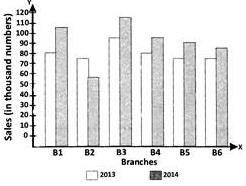What is the average sale of all the branches (in thousand numbers) for the year 2013?

Solution:

The average sales of all the six branches (in thousand numbers) for the year 2013
= 1/6×[80+75+95+85+75+75] = 80.8 = 81

QUESTION: 6

The bar-graph provided gives the sales of books (in thousand numbers) by six branches of a publishing company during two consecutive years 2013 and 2014. Sales of books (in thousand numbers) in different branches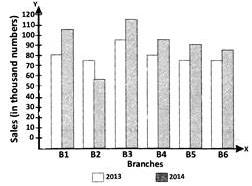What is the ratio of the total sales of branch B2 for both years to the total sales of branch B4 for both the years

Solution:

The required ratio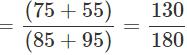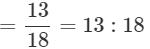QUESTION: 7

The bar-graph provided gives the sales of books (in thousand numbers) by six branches of a publishing company during two consecutive years 2013 and 2014. Sales of books (in thousand numbers) in different branches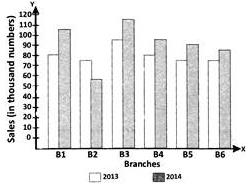What percent of the average sales of branches B1,B2 and B3 in 2014 is the average sales of branches B1,B3 and B6 in 2013?

Solution:

Average sale of B1,B2 and B3 in 2014=(105+115+55)/3=91.667
Average sale of B1,B3 and B6 in 2013=(80+75+95)/3=83.333
83.333=x*91.667/100
x=8333.3/91.667=91(Approx.)

QUESTION: 8

Direction: The following pie charts show the distribution of students of graduate and post-graduate levels in seven different institutes M, N , P, Q, R, S and T town.

Total number of students of graduate level = 27300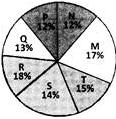Total number of students of post-graduate level = 24700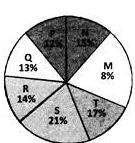Note: Pie charts are not drawn to scale. How many students of institutes M and S are studying at graduate level?

Solution:

Students of institute M at graduate level = 17% of 27300 =4641 Students of institute S at graduate level = 14 % of 27300 = 3822

∴ Total number of students at graduate level in institutes M and S = 4641+3822 = 8463 (or) 31% of 27300 = 8463

QUESTION: 9

The following pie charts show the Jistribution of students of graduate and 3ost-graduate levels in seven different institutes M, M P, Q, R, S and Tina town. Distribution of students at graduate and post-graduate levels in seven institutes M, N, P,Q, R, S, and T. Total number of students of graduate level = 27300Total number of students of post-graduate level = 24700Note: Pie charts are not drawn to scale. What is the total number of students studying at post-graduate level from institutes N and P?

Solution:
QUESTION: 10

The following pie charts show the Jistribution of students of graduate and 3ost-graduate levels in seven different institutes M, M P, Q, R, S and Tina town. Distribution of students at graduate and post-graduate levels in seven institutes M, N, P,Q, R, S, and T. Total number of students of graduate level = 27300Total number of students of post-graduate level = 24700Note: Pie charts are not drawn to scale. What is the total number of graduate and post-graduate level students in the institute R?

Solution:
QUESTION: 11

The following pie charts show the Jistribution of students of graduate and 3ost-graduate levels in seven different institutes M, M P, Q, R, S and Tina town. Distribution of students at graduate and post-graduate levels in seven institutes M, N, P,Q, R, S, and T. Total number of students of graduate level = 27300Total number of students of post-graduate level = 24700Note: Pie charts are not drawn to scale. What is the total number of graduate and post-graduate level students in the institute R?

Solution:
QUESTION: 12

The following pie charts show the Jistribution of students of graduate and 3ost-graduate levels in seven different institutes M, M P, Q, R, S and Tina town. Distribution of students at graduate and post-graduate levels in seven institutes M, N, P,Q, R, S, and T. Total number of students of graduate level = 27300Total number of students of post-graduate level = 24700Note: Pie charts are not drawn to scale. What is the total number of graduate and post-graduate level students in the institute R?

Solution:
QUESTION: 13

The bar chart shows the number of workers employed by a trading company in three years.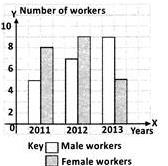Calculate the difference between the total number of male and female workers employed over those three years.

Solution:

The total number of male workers employed = 5+7+9 = 21
The total number of female workers employed =8+9+5 = 22
∴ The required difference = 22−21 = 1

QUESTION: 14

The line graph shows the sale of dolls by Suhas from Monday to Saturday in a particular week.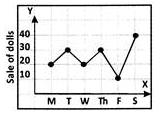Given that the cost of one doll is Rs.35, how much did Suhas receive from the sale or dolls on Saturday?

Solution:

Sale of dolls on Saturday = 40 Cost of each doll = Rs.35
∴ Amount earned Rs.35 × 40 = Rs.1400

QUESTION: 15

The bar chart shows the number of 'Maruti' and 'Benz' cars sold by three dealers, A, B and C.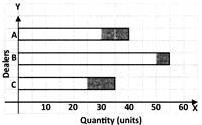Which of the following statements is true?

Solution:

The number of Benz cars sold by dealer B is the maximum.

QUESTION: 16

The line graph shows the price of chicken in towns M and N.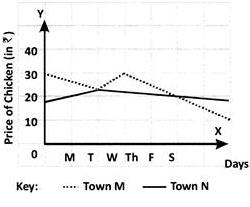On which days are the price of chicken the same in both the towns?

Solution:

The price of chicken is the same in both the towns ⇒ their graphs intersect at a point. This happens to be on Tuesday and Saturday.

QUESTION: 17

The line graph shows the sale of watches in a company.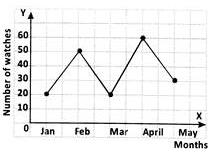How many watches were sold in all 5 months?

Solution:
QUESTION: 18

Direction: The line graph shows the monthly expenditure of Vasu's family.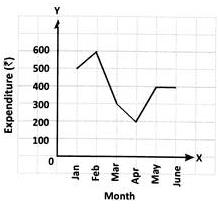What is the total expenditure over the first 3 months?

Solution:
QUESTION: 19

Direction: The line graph shows the monthly expenditure of Vasu's family.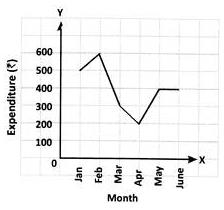Find the difference between their highest and lowest monthly expenditures.

Solution:
QUESTION: 20

Which of the following statements is correct?

Solution: